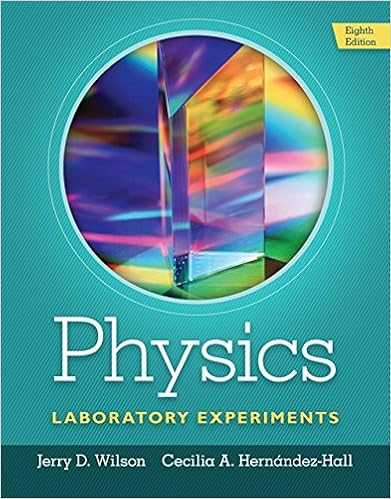# lab report # 3 vector addition.docx - PHYS 2125 UNIVERSITY...

• Lab Report
• 7
• 75% (4) 3 out of 4 people found this document helpful

This preview shows page 1 - 3 out of 7 pages.

##### We have textbook solutions for you!
The document you are viewing contains questions related to this textbook.The document you are viewing contains questions related to this textbook.
Chapter 12 / Exercise 1
Physics Laboratory Experiments
WilsonExpert Verified
PHYS 2125: UNIVERSITY PHYSICS I LAB.LAB REPORT, EXPERIMENT # 3Experiment date: 10/04/2018Title:VECTOR ADDITIONPresented by:CHARLES KENGNE CHOUTEDJEMInstructor:Dr. C. DEL CERRO
##### We have textbook solutions for you!
The document you are viewing contains questions related to this textbook.The document you are viewing contains questions related to this textbook.
Chapter 12 / Exercise 1
Physics Laboratory Experiments
WilsonExpert Verified
InstructionsObjectives of the experiment:Experimentally verify the laws of addition of vectors by using both the geometrical and the analytical methods.Equipment Needed:1. Force table 5. Strings for suspending the masses2. Weight holders (#4). 6. A ring3. Pulleys (#4). 7. A metal pin4. Slotted weights 8. A protractor9. Sheets of plain paper and graph paper.Theory:A vector A can be written as a sum of two vectors Ax and Ay along the x and the y-axis respectively, as shown. Ax and Ay are called the components of vector A and are given byAx = A cosαAy = A sinα α (≤ 900) is the angle vector A makes with the x-axis.In order to find the resultant vector R of a system of vectors A, B, C, etc we follow these steps:a) Find the x and y components for each vector using the above equations. i.e find:
R is :
•••